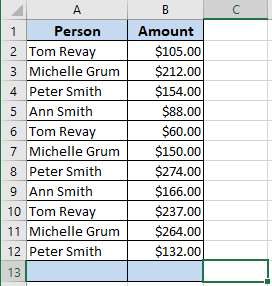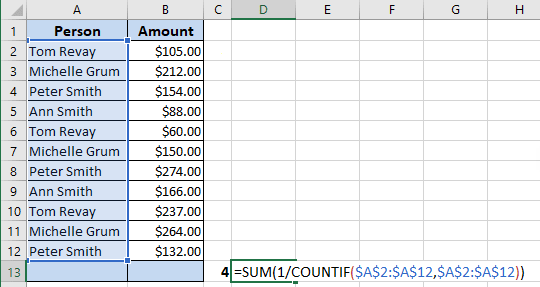# Counting the number of unique values

Excel
You can easily count the number of unique values of the range using a simple formula.

For example, you want to count the number of unique values of the range:You can use the next formula:

= SUM (1 / COUNTIF (\$A\$2:\$A\$12, \$A\$2:\$A\$12)):This formula in Excel for Microsoft 365 has no limitation: If the range contains any blank cells, some previous versions of Excel return an error.

See also this tip in French: Compter le nombre de valeurs uniques.